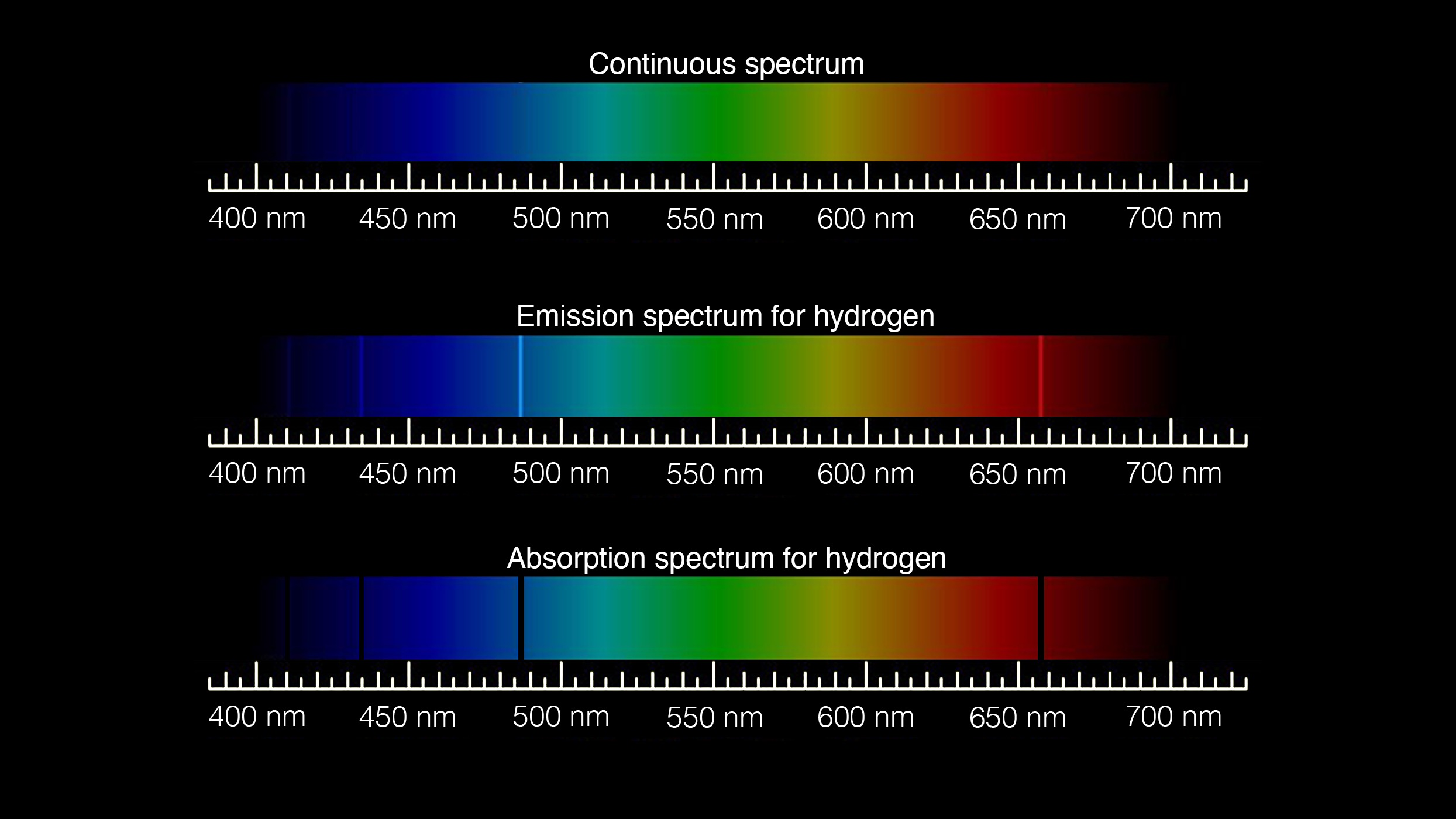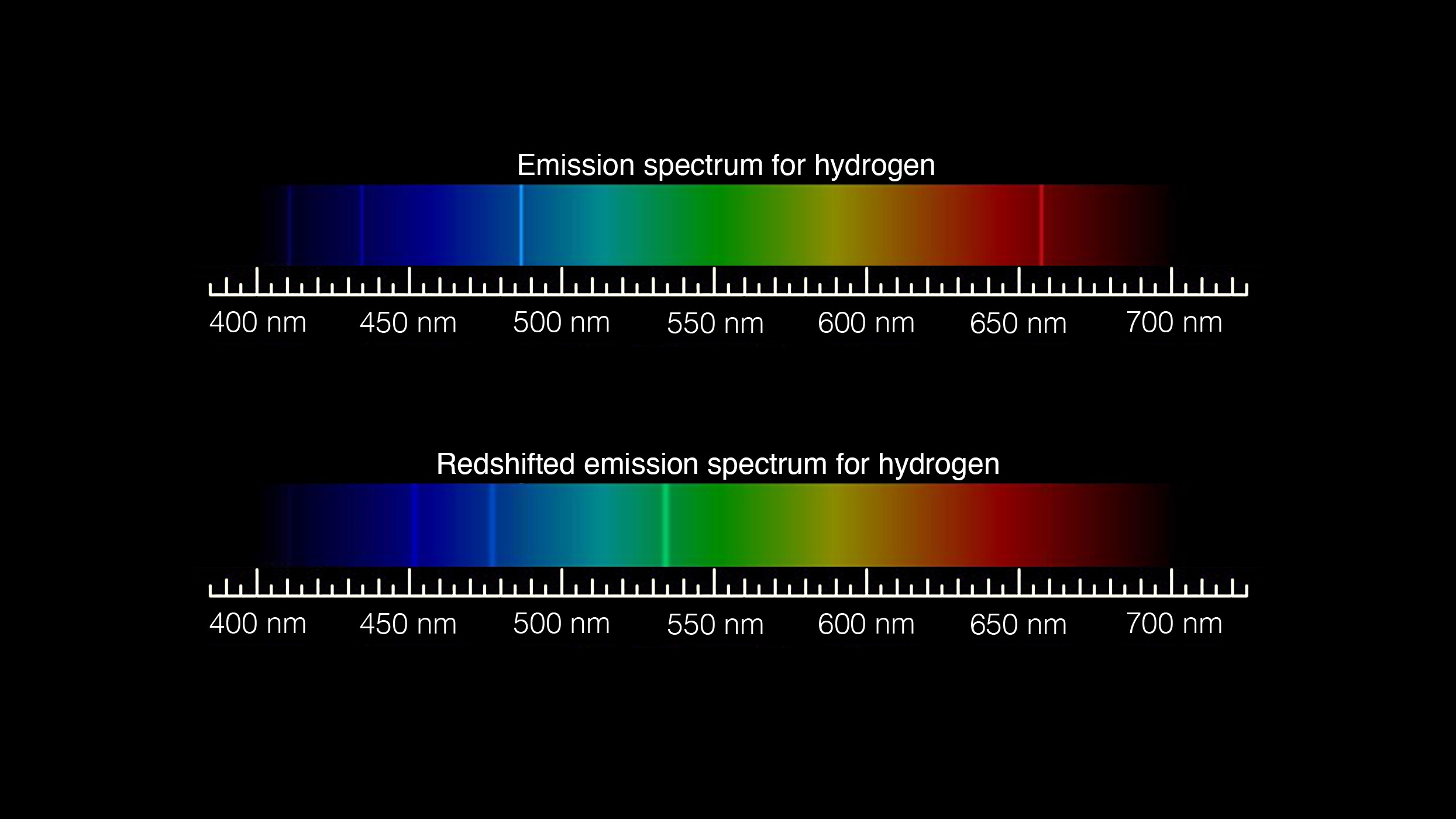Activity Notes

### Overview

In this activity, students will learn about the expanding universe and the redshift of lightwaves. They will then calculate the redshift of a supernova, determine its velocity relative to Earth, and find the distance to that object.

### Management

• Students should have a general understanding of the electromagnetic spectrum and/or wave properties (i.e., amplitude, wavelength, frequency, and speed).

#### Tips for Remote Instruction

• Use an overhead camera to show students the stretching elastic demo. Place a ruler parallel to the elastic so students can see the numeric change in distance between wave crests.

### Background

#### Understanding redshift

Our eyes can only see a small portion of the electromagnetic spectrum – the portion we call visible light. Other portions of the spectrum have wavelengths too long or too short for our eyes to perceive.Visible light, the type of light humans can perceive with their eyes, is just one tiny piece of the electromagnetic spectrum. This chart compares the wavelength and frequency range of each kind of wave on the electromagnetic spectrum. Note: The graphic representations are not to scale. Image credit: NASA/JPL-Caltech | + Expand image | › Download low-ink version for printing

Typically, the human eye can detect wavelengths from 380 to 700 nanometers (nm). For comparison, a human hair is about 80,000 nm wide. As the full spectrum of visible light travels through a prism, the wavelengths separate into the colors of the rainbow because each color is a different wavelength, thus refracting, or bending, a different amount. Violet has the shortest wavelength at around 380 nanometers and red has the longest wavelength at around 700 nanometers.

Every type of atom or molecule gives off energy at specific wavelengths. For example, hydrogen atoms emit visible light at 410.2 nm, 434.0 nm, 486.1 nm, and 656.3 nm. This predictable pattern of emitted light is called an emission spectrum.This graphic shows the difference between a continuous spectrum and emission and absorption spectra for the element hydrogen. An emission spectrum shows bright lines at the wavelengths where light is emitted from atoms and molecules in a hot gas. An absorption spectrum shows dark lines at the wavelengths where light is absorbed when passing through gaseous elements in a star’s atmosphere. Image credit: NASA/JPL-Caltech | + Expand image

Close examination of the visible-light spectrum from our Sun and other stars reveals spectral lines that act like fingerprints for atoms and molecules. Identifying these patterns tells scientists what elements and molecules make up a star.

Unlike light leaving the Sun, which takes 8.5 minutes to reach Earth, light leaving distant stars and galaxies can take billions of years to reach our planet. As they travel, the waves that make up the light get stretched as the universe expands – something it’s been doing since the Big Bang.

What this means for light coming from distant galaxies moving away from Earth as the universe expands is that the visible lightwaves you would be able to see with your eyes get stretched into longer wavelengths and shift from visible light toward infrared.

In the case of hydrogen, the four emission lines get shifted toward the redder (longer wavelength) end of the electromagnetic spectrum. Scientists refer to this phenomenon as redshift – and the farther away an object is, the more redshift it undergoes.Notice how the bright lines on the standard emission spectrum for hydrogen (top) have moved toward the red end of the spectrum on the redshifted emission spectrum (bottom). Image credit: NASA/JPL-Caltech | + Expand image

#### Calculating redshift

Redshift is given a value (z) and is calculated with the following equation:

z = (Wavelengtho - Wavelengthr) / Wavelengthr

z = redshift
Wavelengthr = the known wavelength of an element not experiencing redshift
Wavelengtho = the observed wavelength of that emission line

By determining the redshift of an object, scientists can calculate the speed at which the object is moving away from us (as a result of the universe expanding) and the distance to the object from Earth.

Missions such as the James Webb Space Telescope take advantage of this phenomenon by viewing light in the near- and mid-infrared wavelengths – the wavelengths to which light has shifted after traveling from the most distant, and therefore oldest, stars and galaxies. This allows astronomers to peer into the early history of the universe.

### Procedures

1. Build or activate students’ background knowledge by discussing the electromagnetic spectrum, different types of radiation, and wave properties.
2. Explain to students the concept of the expanding universe. Impress that space-time, the fabric of the universe itself, is expanding – it's not just that objects within the universe are moving apart. Consider using the analogy of a loaf of raisin bread expanding as it bakes, with the dough representing space-time and the raisins representing galaxies or galaxy clusters.

Alternatively, draw several dots on a balloon with a felt pen. Explain to students that the balloon represents space-time, and the dots represent galaxies or galaxy clusters. Ask them to predict what will occur when the balloon inflates. (As the balloon expands, the distance between the dots will expand as well.)
3. Complete one or both of the modeling and calculating sections with students. If completing some or all of the calculations, students should complete them in order.

#### Model redshift

Either as a class demonstration or in groups of 2-3 students, use elastic bands as follows to model the expansion of spacetime and the stretching of visible light into the infrared.

1. Have students use a felt pen and a ruler to mark evenly spaced dots on the elastic to represent the crest of a visible light wave. Have students make note of how far apart their dots are.Draw evenly spaced dots on a strip of elastic. Image credit: NASA/JPL-Caltech | + Expand image

For added complexity, tell students that for this model, 1 millimeter on the elastic represents 100 nm and to place their dots five or six mm apart from each other. Ask students to identify the color in the visible spectrum that their wavelength represents.

In either case, if needed for students to visualize a wave, they can draw in the rest of the wave pattern on the elastic.Optionally, draw wave segments connecting the dots. Image credit: NASA/JPL-Caltech | + Expand image

2. Have one student slowly stretch the elastic and hold it at its stretched length. Students can opt to stretch their elastic as far as it will go, or only stretch it a small amount. With the elastic stretched, another student should measure the distance between the dots (or wave crests) and record the distance.The wavelength drawn on the piece of elastic gets longer as the elastic is stretched. Image credit: NASA/JPL-Caltech | + Expand image

3. Groups should identify that the distance between the dots or crests increased.

If students used the scale measurements and identified the light color in Step 1, they should now convert their elastic measurements to scaled nanometer measurements and identify where on the electromagnetic spectrum their stretched wave is located.

#### Calculate redshift

Students will use the redshift equation, emission spectrum of hydrogen, and spectra from supernova iPTF15th to calculate the redshift of the supernova.

1. Introduce students to the equation used to calculate redshift:

z = (Wavelengtho - Wavelengthr) / Wavelengthr

z = redshift
Wavelengthr = the known wavelength of an element not experiencing redshift
Wavelengtho = the observed wavelength of that emission line

Explain that by calculating the redshift value students will be able to determine the velocity at which an object is moving away from Earth as a result of the expansion of the galaxy. With that information, they can then determine the distance to that object.

2. Have students calculate the redshift for one of the emission lines below, then compare the results as a class.Location of Hydrogen Spectral Lines at Rest (placed over spectrum from supernova iPTF15th). Image credit: WISeREP (2012PASP..124..668Y) | + Expand image

Location of hydrogen spectral lines at rest:
Line 1: 410.2 nm
Line 2: 434.0 nm
Line 3: 486.1 nm
Line 4: 656.3 nmLocation of Hydrogen Spectral Lines Observed (in spectrum from supernova iPTF15th). Image credit: WISeREP (2012PASP..124..668Y) | + Expand image

Location of hydrogen spectral lines observed in spectrum from supernova iPTF15th:
Line 1: 4511.91 Å
Line 2: 4773.69 Å
Line 3: 5347.64 Å
Line 4: 7219.33 ÅLocation of Hydrogen Spectral Lines - At Rest vs. Observed (in spectrum from supernova iPTF15th). Image credit: WISeREP (2012PASP..124..668Y) | + Expand image

Line 1: z = 0.09993
Line 2: z = 0.0999
Line 3: z = 0.1001
Line 4: z = 0.1000

3. Next, have them find the average redshift value for all four lines.

#### Calculate velocity

Students will use the Doppler shift formula to find the velocity of supernova iPTF15th away from Earth.

1. Introduce students to the Doppler shift formula:

((wavelengtho - wavelengthr)/wavelengthr) = v/c

v = velocity
c = the speed of light in a vacuum (3 x 108 m/sec)

2. Have students use the Doppler shift formula to find the velocity of supernova iPTF15th away from Earth. If necessary, remind students that half of this equation was solved in the previous step when finding the average z value.

Answer: 0.1 * c = v => v = 3 x 107 m/sec

#### Calculate distance

Students will use Hubble's Law to find the distance to supernova iPTF15th.

1. Introduce students to Hubble’s Law, which is the observation that galaxies are moving away from Earth at speeds proportional to their distance (d) and is expressed as:

v = H0 * d

v = velocity
H0 = the Hubble constant of approximately 70 km/sec/Megaparsec
d = distance

Note: A parsec is a unit of distance equal to approximately 3.26 light years, and a Megaparsec is equal to one million parsecs.

2. Have students use Hubble’s Law to find the distance to supernova iPTF15th.

Answer: One Megaparsec (Mpc) = 3.26 * 106 light year.
3 * 107 m/s = 70 km/s/Mpc * d
d = 3 * 107 m/s / 7 * 104 m/s/Mpc
d ≈ 429 Mpc
429 Mpc ≈ 1.4 x 109 light years

### Discussion

• What implications does redshift have for making observations of the most distant stars and galaxies, where even ultraviolet light may have redshifted beyond the visible spectrum?
• If light has been traveling from a star, supernova or galaxy for one billion years, does that mean the object is currently one billion light years away?

Answer: The object would be farther away since the universe is expanding and the object has moved away from Earth during the time the light has been traveling toward Earth.
• Do you think all objects in the universe are redshifted?

Answer: As the universe expands galaxy clusters and other distant objects are moving apart from each other, displaying redshift. However, nearby objects, such as planets, and even local galaxies, are gravitationally bound and not displaying redshift as a result of the galaxy expanding. In fact, the Milky Way and the Andromeda galaxies are moving toward each other.
• What would you expect to see if the universe was contracting rather than expanding?

Answer: Students would expect to see blue shifted wavelengths, as the light traveling toward the observer on Earth was compressed into shorter wavelengths as the space it was traveling through contracted.

### Assessment

• Students should correctly measure a longer wavelength on the stretched elastic.
• Students should calculate the correct z, v and d values in the problem set.